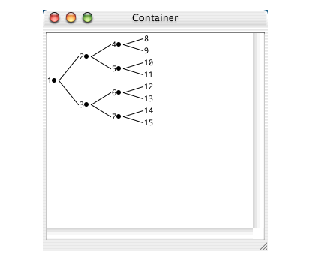### 4.3 Graph panes

Another kind of choice is the ``` graph-pane``` . This is a special pane that can draw graphs, whose nodes and edges can be selected, and for which callbacks can be specified, as usual.

Here is a simple example of a graph pane. It draws a small rooted tree:

`(contain `
` (make-instance`
`  'graph-pane`
`  :roots '(1)`
`  :children-function `
`  #'(lambda (x)`
`      (when (< x 8)`
`       (list (* 2 x) (1+ (* 2 x))))))) `

Figure 4.7 A graph paneThe graph pane is supplied with a ``` :children-function``` which it uses to calculate the children of the root node, and from those children it continues to calculate more children until the termination condition is reached. For more details of this, see the LispWorks CAPI Reference Manual .

``` graph-pane``` provides a gesture which expands or collapses a node, depending on it current state. Click on the circle alongside the node to expand or collapse it.

You can associate selection, retraction, extension, and action callbacks with any or all elements of a graph. Here is a simple graph pane that has an action callback on its nodes.

First we need a pane for displaying the callback messages in. This is done by executing the following code:

`(defvar *the-collector*`
`   (contain (make-instance 'collector-pane)))`

Then, define the following four callback functions:

`(defun test-action-callback (&rest args)`
`            (format (collector-pane-stream `
`                     *the-collector*) "Action"))`
`(defun test-selection-callback (&rest args)`
`            (format (collector-pane-stream *the-collector*)`
`                    "Selection"))`
`(defun test-extend-callback (&rest args)`
`            (format (collector-pane-stream *the-collector*)`
`                    "Extend"))`
`(defun test-retract-callback (&rest args)`
`            (format (collector-pane-stream *the-collector*)`
`                    "Retract"))`

Now create an extended selection graph pane which uses each of these callbacks, the callback used depending on the action taken:

`(contain`
`   (make-instance`
`      'graph-pane `
`      :interaction :extended-selection`
`      :roots '(1)`
`      :children-function`
`       #'(lambda (x)`
`            (when (< x 8)`
`                  (list (* 2 x) (1+ (* 2 x)))))`
`      :action-callback 'test-action-callback`
`      :selection-callback 'test-selection-callback`
`      :extend-callback 'test-extend-callback`
`      :retract-callback 'test-retract-callback))`

The selection callback function is called whenever any node in the graph is selected.

The extension callback function is called when the selection is extended by middle clicking on another node (thus selecting it too).

The retract callback function is called whenever an already selected node is deselected.

The action callback function is called whenever an action is performed on a node (that is, whenever it gets a double-click, or Return is pressed while the node is selected).

LispWorks CAPI User Guide (Macintosh version) - 8 Apr 2005